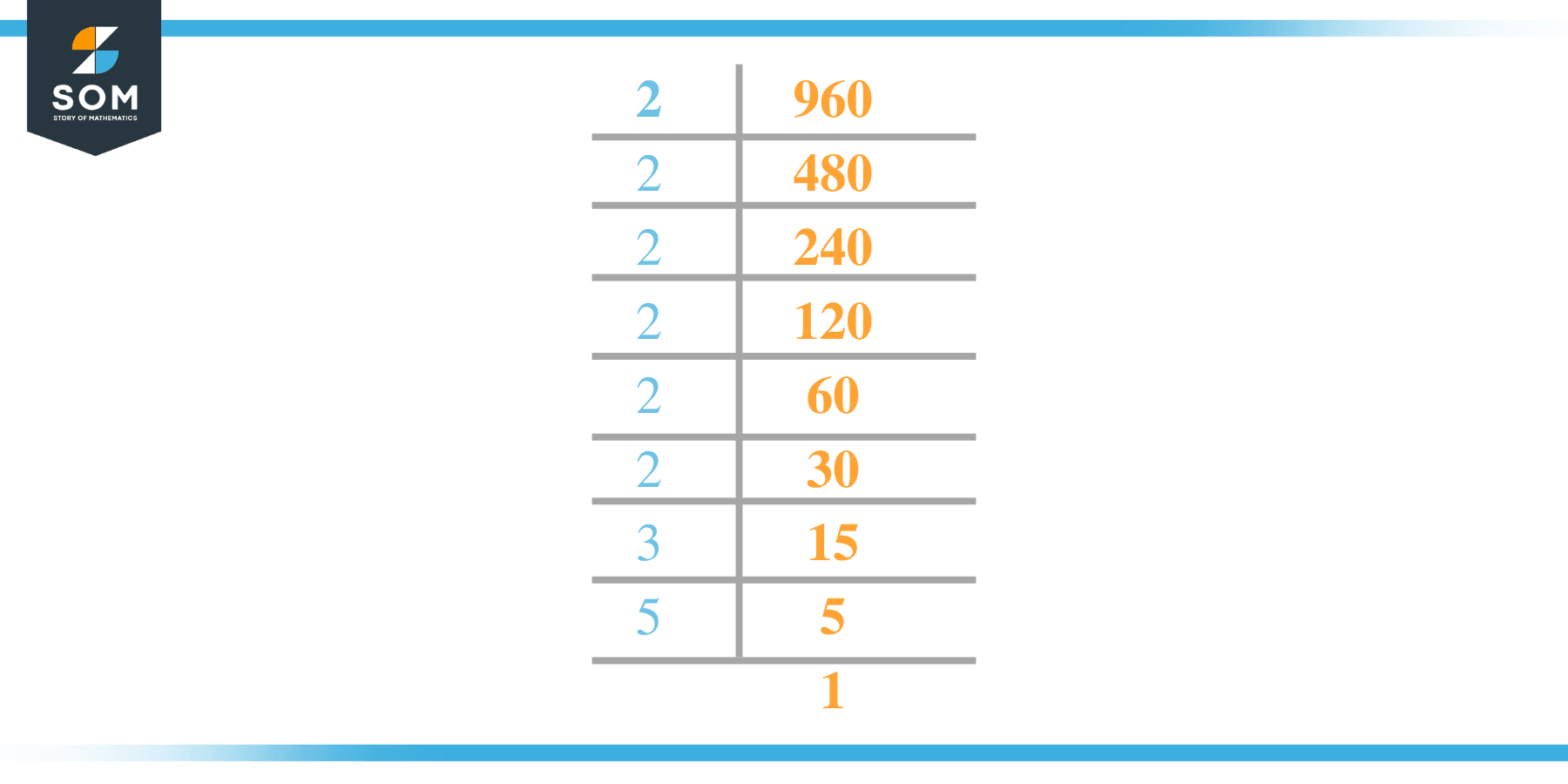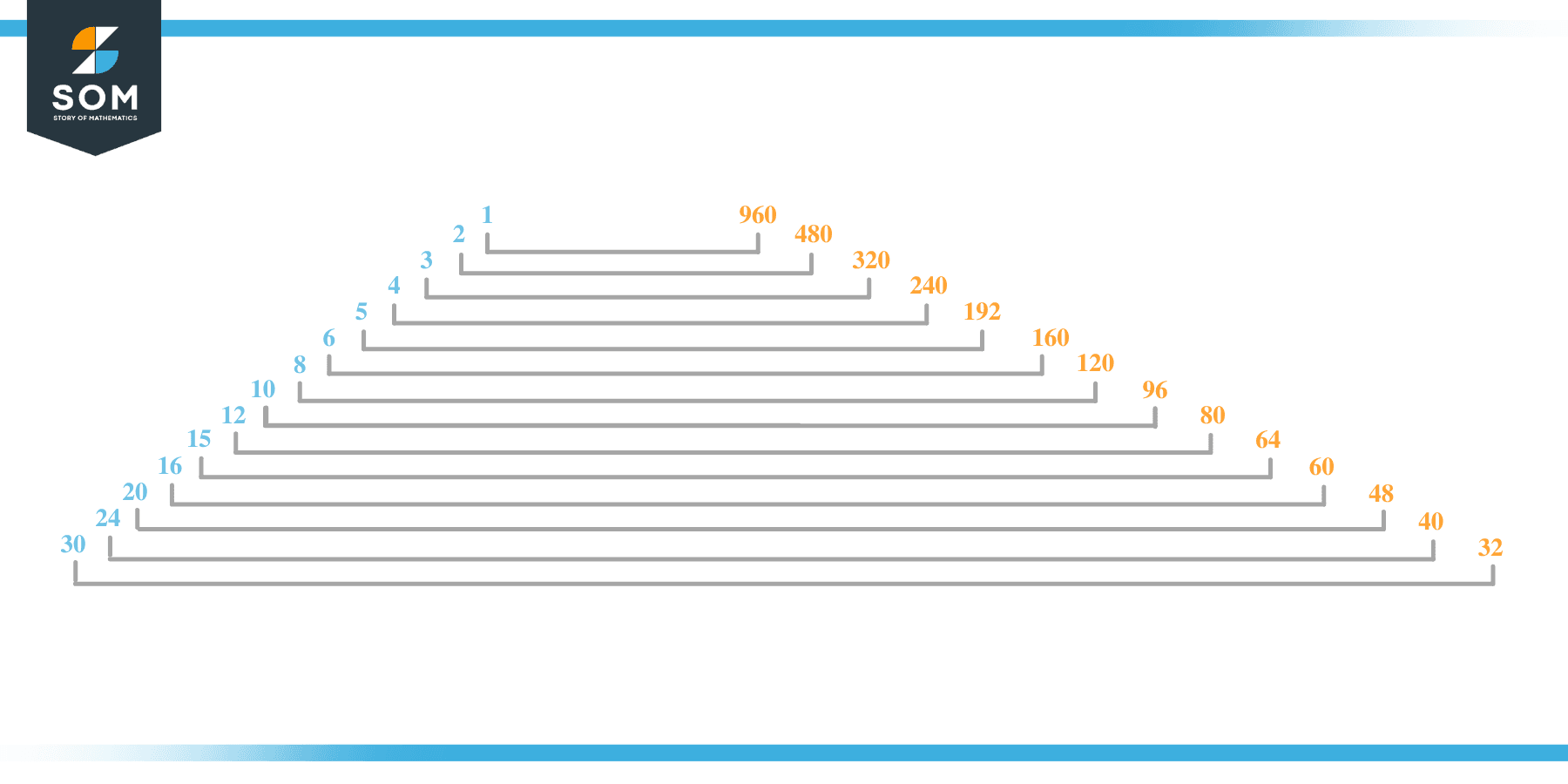# Factors of 960: Prime Factorization, Methods, and Examples

There are 28 positive factors of number 960 and the same number are negative ones so in total it has 56 factors which discuss below in detail. These factors when placed in the denominator and number960 in the numerator then the result is a whole number which shows it is a factor.As the 960 number is concerned so it is an even composite number.

### Factors of 960

Here are the factors of number 960.

Factors of 960: 1, 2, 3, 4, 5, 6, 8, 10, 12, 15, 16, 20, 24, 30, 32, 40, 48, 60, 64, 80, 96, 120, 160, 192, 240, 320, 480, and 960

### Negative Factors of 960

The negative factors of 960 are similar to its positive aspects, just with a negative sign.

Negative Factors of 960: -1, -2, -3, -4, -5, -6, -8, -10, -12, -15, -16, -20, -24, -30, -32, -40, -48, -60, -64, -80, -96, -120, -160, -192, -240, -320, -480, and -960

### Prime Factorization of 960

The prime factorization of 960 is the way of expressing its prime factors in the product form.

Prime Factorization: 2 x 2 x 2 x 2 x 2 x 2 x 3 x 5

In this article, we will learn about the factors of 960 and how to find them using various techniques such as upside-down division, prime factorization, and factor tree.

## What Are the Factors of 960?

The factors of 960 are 1, 2, 3, 4, 5, 6, 8, 10, 12, 15, 16, 20, 24, 30, 32, 40, 48, 60, 64, 80, 96, 120, 160, 192, 240, 320, 480, and 960. These numbers are the factors as they do not leave any remainder when divided by 960.

The factors of 960 are classified as prime numbers and composite numbers. The prime factors of the number 960 can be determined using the prime factorization technique.

## How To Find the Factors of 960?

You can find the factors of 960 by using the rules of divisibility. The divisibility rule states that any number, when divided by any other natural number, is said to be divisible by the number if the quotient is the whole number and the resulting remainder is zero.

To find the factors of 960, create a list containing the numbers that are exactly divisible by 960 with zero remainders. One important thing to note is that 1 and 960 are the960’s factors as every natural number has 1 and the number itself as its factor.

1 is also called the universal factor of every number. The factors of 960 are determined as follows:

$\dfrac{960}{1} = 960$

$\dfrac{960}{2} = 480$

$\dfrac{960}{3} = 320$

$\dfrac{960}{4} = 240$

$\dfrac{960}{5} = 192$

$\dfrac{960}{6} = 160$

$\dfrac{960}{8} = 120$

$\dfrac{960}{10} = 96$

$\dfrac{960}{12} = 80$

$\dfrac{960}{15} = 64$

$\dfrac{960}{16} = 60$

$\dfrac{960}{20} = 48$

$\dfrac{960}{24} = 40$

$\dfrac{960}{30} = 32$

$\dfrac{960}{32} = 30$

$\dfrac{960}{960} = 1$

Therefore, 1, 2, 3, 4, 5, 6, 8, 10, 12, 15, 16, 20, 24, 30, 32, 40, 48, 60, 64, 80, 96, 120, 160, 192, 240, 320, 480, and 960 are the factors of 960.

### Total Number of Factors of 960

For 960, there are 28 positive factors and 28 negative ones. So in total, there are 56 factors of 960.

To find the total number of factors of the given number, follow the procedure mentioned below:

1. Find the factorization/prime factorization of the given number.
2. Demonstrate the prime factorization of the number in the form of exponent form.
3. Add 1 to each of the exponents of the prime factor.
4. Now, multiply the resulting exponents together. This obtained product is equivalent to the total number of factors of the given number.

By following this procedure, the total number of factors of 960 is given as:

Factorization of 960 is 2 x 2 x 2 x 2 x 2 x 2 x 3 x 5.

The exponent of 2, 3, and 5 is 6, 1,1.

Adding 1 to each and multiplying them together results in 28.

Therefore, the total number of factors of 960 is 56. Twenty-eight are positive, and twenty-eight factors are negative.

### Important Notes

Here are some essential points that must be considered while finding the factors of any given number:

• The factor of any given number must be a whole number.
• The factors of the number cannot be in the form of decimals or fractions.
• Factors can be positive as well as negative.
• Negative factors are the additive inverse of the positive factors of a given number.
• The factor of a number cannot be greater than that number.
• Every even number has 2 as its prime factor, the smallest prime factor.

## Factors of 960 by Prime FactorizationThe number 960 is a composite. Prime factorization is a valuable technique for finding the number’s prime factors and expressing the number as the product of its prime factors.

Before finding the factors of 960 using prime factorization, let us find out what prime factors are. Prime factors are the factors of any given number that are only divisible by 1 and themselves.

To start the prime factorization of 960, start dividing by its most minor prime factor. First, determine that the given number is either even or odd. If it is an even number, then 2 will be the smallest prime factor.

Continue splitting the quotient obtained until 1 is received as the quotient. The prime factorization of 960 can be expressed as:

960 = 2 x 2 x 2 x 2 x 2 x 2 x 3 x 5

## Factors of 960 in PairsThe factor pairs are the duplet of numbers that, when multiplied together, result in the factorized number. Factor pairs can be more than one depending on the total number of factors given.

For 960, the factor pairs can be found as:

1 x 960 = 960

2 x 480 = 960

3 x 320 = 960

4 x 240 = 960

5 x 192 = 960

6 x 160 = 960

8 x 120 = 960

10 x 96 = 960

12 x 80 = 960

15 x 64 = 960

16 x 60 = 960

20 x 48 = 960

24 x 40 = 960

30 x 32 = 960

The possible factor pairs of 960 are given as (1, 960), (2, 480), (3, 320), (4, 240), (5, 192), (6, 160), (8, 120),(10, 96), (12, 80), (15, 64), (16, 60), (20, 48), (24, 40) and (30, 32) .

All these numbers in pairs, when multiplied, give 960 as the product.

The negative factor pairs of 960 are given as:

-1 x -960 = 960

-2 x -480 = 960

-3 x -320 = 960

-4 x -240 = 960

-5 x -192 = 960

-6 x -160 = 960

-8 x -120 = 960

-10 x -96 = 960

-12 x -80 = 960

-15 x -64 = 960

-16 x -60 = 960

-20 x -48 = 960

-24 x -40 = 960

-30 x -32 = 960

It is important to note that in negative factor pairs, the minus sign has been multiplied by the minus sign, due to which the resulting product is the original positive number. Therefore, -1, -2, -3, -4, -5, -6, -8, -10, -12, -15, -16, -20, -24, -30, -32, -40, -48, -60, -64, -80, -96, -120, -160, -192, -240, -320, -480, and -960 are called negative factors of 960.

The list of all the factors of 960, including positive as well as negative numbers, is given below.

Factor list of 960: 1, -1, 2, -2, 3, -3, 4, -4, 5, -5, 6, -6, 8, -8, 10, -10, 12, -12, 15, -15, 16, -16, 20, -20, 24, -24, 30, -30, 32, -32, 40, -40, 48, -48, 60, -60, 64, -64, 80, -80, 96, -96, 120, -120, 160, -160, 192, -192, 240, -240, 320, -320, 480, -480,  960 and -960

## Factors of 960 Solved Examples

To better understand the concept of factors, let’s solve some examples.

### Example 1

How many factors of 960 are there?

### Solution

The total number of Factors of 960 is 28.

Factors of 960 are 1, 2, 3, 4, 5, 6, 8, 10, 12, 15, 16, 20, 24, 30, 32, 40, 48, 60, 64, 80, 96, 120, 160, 192, 240, 320, 480, and 960

### Example 2

Find the factors of 960 using prime factorization.

### Solution

The prime factorization of 960 is given as:

960 $\div$ 2 = 480

480 $\div$ 2 = 240

240 $\div$ 2 = 120

120 $\div$ 2 = 60

60 $\div$ 2 = 30

30 $\div$ 2 = 15

15 $\div$ 3 = 5

5 $\div$ 5 = 1

So the prime factorization of 960 can be written as:

2 x 2 x 2 x 2 x 2 x 2 x 3 x 5 = 960